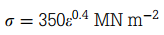### Create an Account

Home / Questions / The curve of true stress against true strain for the metal wire approximates to Estimate ...

# The curve of true stress against true strain for the metal wire approximates to Estimate the tensile strength of the wire and the work required to take 1 m3 of the wire to the point of necking

1. The curve of true stress against true strain for the metal wire approximates toEstimate the tensile strength of the wire and the work required to take 1 m3 of the wire to the point of necking

2. a. If the true stress–true strain curve for a material is defined by σ = Aεn, where A and n are constants, find the tensile strength sTS.

b. For a nickel alloy, n = 0.2 and A = 800 MN m-2. Evaluate the tensile strength of the alloy. Evaluate the true stress in an alloy specimen loaded to σTS.

May 16 2020 View more View LessSubscribe To Get Solution